### IMO Shortlist 1987 problem 19

Kvaliteta:
Avg: 0,0
Težina:
Avg: 0,0
Let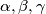$\alpha,\beta,\gamma$ be positive real numbers such that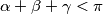$\alpha+\beta+\gamma < \pi$,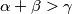$\alpha+\beta > \gamma$,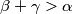$\beta+\gamma > \alpha$,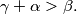$\gamma + \alpha > \beta.$ Prove that with the segments of lengths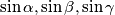$\sin \alpha, \sin \beta, \sin \gamma$ we can construct a triangle and that its area is not greater than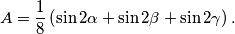Proposed by Soviet Union
Izvor: Međunarodna matematička olimpijada, shortlist 1987